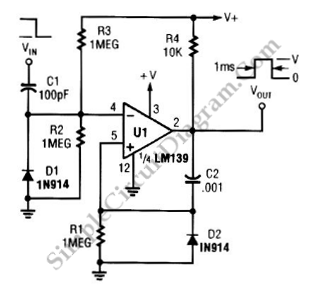# LM139 One-Shot Multivibrator

The circuit below is a one-shot multivibrator. One shot multivibrator is also known as monostable multivibrator, or timer. The main function of such circuit is producing a fixed width pulse. The width of this pulse is determined by the component value selection at the time of designing the circuit. The main component of this circuit is the LM139, and this circuit only uses one section of quad LM139. The pulse width of this circuit is determined by the value of C2 and R1. When selecting the R1 value, it should be more than 10 times of R4 to avoid loading effect. Here is the schematic diagram of the circuit: